# 用Python快速分析、可视化和预测股票价格

Pandas Matplotlib Scikit-learn MachineLearning Quantitative-Finance recommend## 加载雅虎财经数据集

Pandas 网络数据阅读器 （Pandas web data reader）是 Pandas 库的一个扩展，用于与大多数最新的金融数据进行通信，包括雅虎财经、谷歌财经、Enigma 等资源。

import pandas as pd
import datetime
from pandas import Series, DataFrame

start = datetime.datetime(2010, 1, 1)

end = datetime.datetime(2017, 1, 11)

df = web.DataReader("AAPL", 'yahoo', start, end)

df.tail()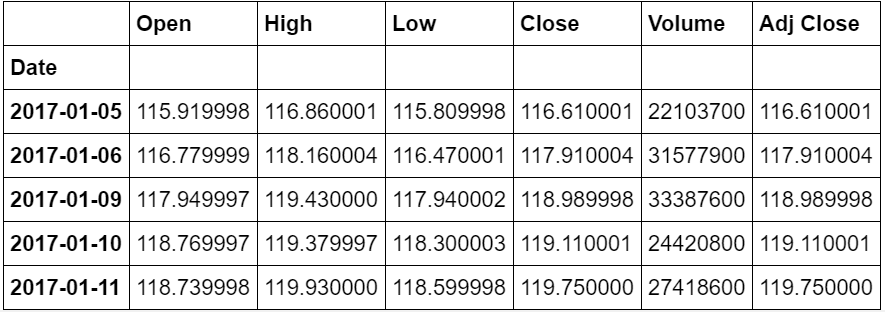## 探索股票的移动平均值和收益率

### 移动平均值：确定趋势

close_px = df['Adj Close']

mavg = close_px.rolling(window=100).mean()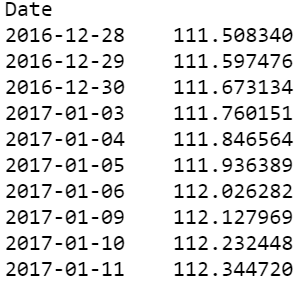%matplotlib inline
import matplotlib.pyplot as plt
from matplotlib import style

# Adjusting the size of matplotlib

import matplotlib as mpl
mpl.rc('figure', figsize=(8, 7))

mpl.__version__

# Adjusting the style of matplotlib

style.use('ggplot')

close_px.plot(label='AAPL')

mavg.plot(label='mavg')

plt.legend()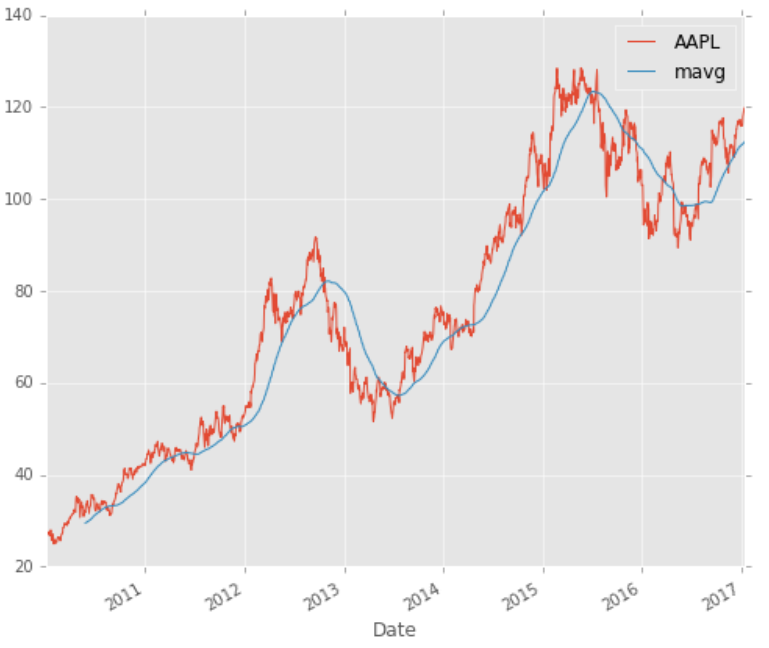### 回报偏差:用于确定风险和收益rets = close_px / close_px.shift(1) - 1

rets.plot(label='return')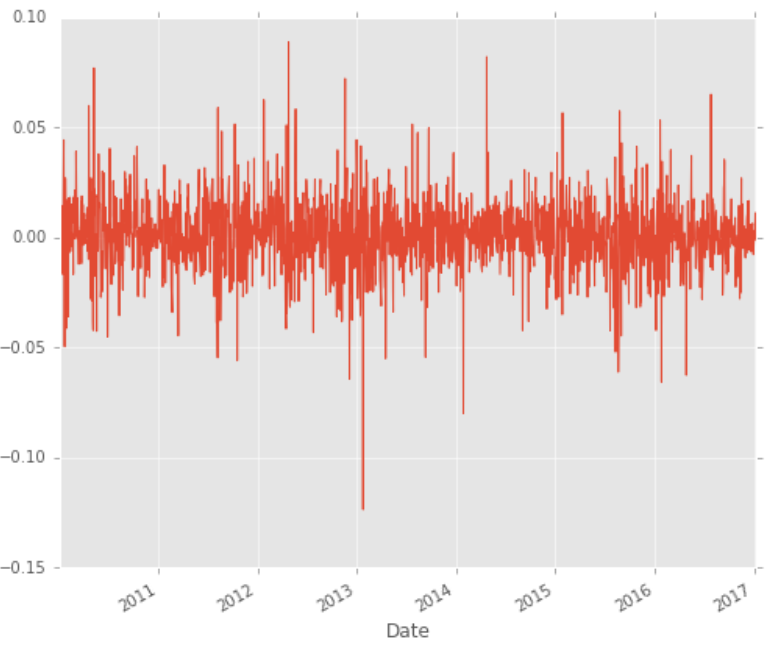## 分析竞争对手股票

dfcomp = web.DataReader(['AAPL', 'GE', 'GOOG', 'IBM', 'MSFT'],'yahoo',start=start,end=end)['Adj Close']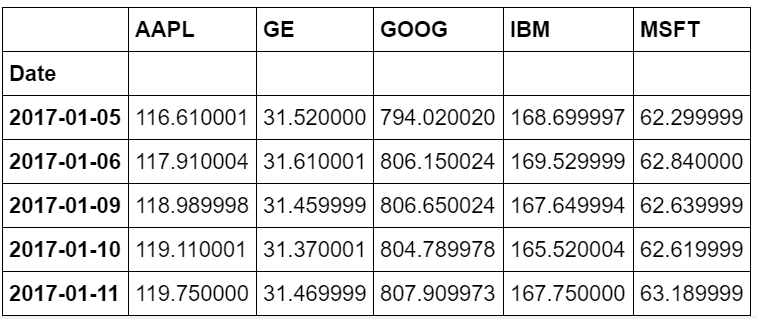### 相关性分析:竞争对手会互相影响吗？

retscomp = dfcomp.pct_change()

corr = retscomp.corr()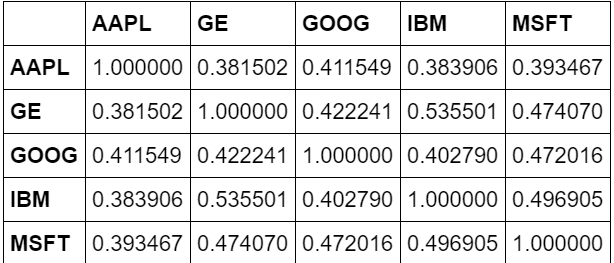plt.scatter(retscomp.AAPL, retscomp.GE)

plt.xlabel('Returns AAPL')

plt.ylabel('Returns GE')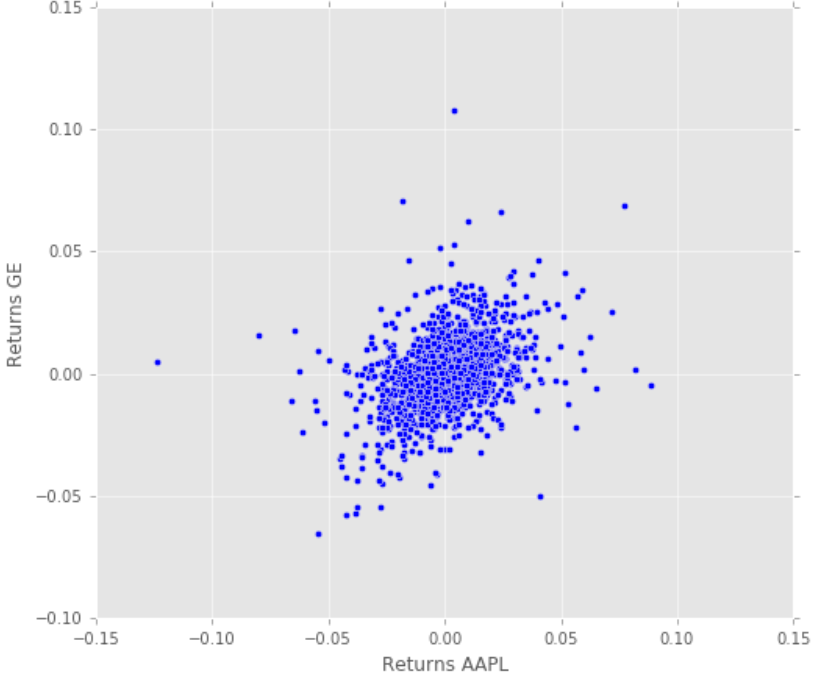Apple 和 GE 的散点图

pd.scatter_matrix(retscomp, diagonal='kde', figsize=(10, 10))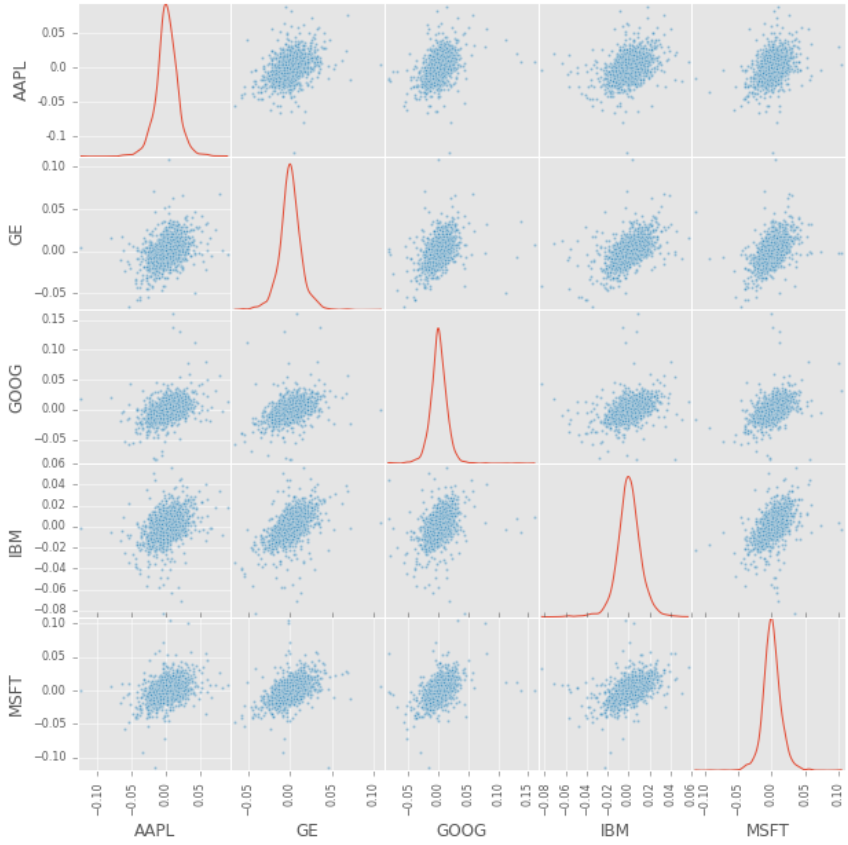KDE 图和散点矩阵

plt.imshow(corr, cmap='hot', interpolation='none')
plt.colorbar()

plt.xticks(range(len(corr)), corr.columns)
plt.yticks(range(len(corr)), corr.columns)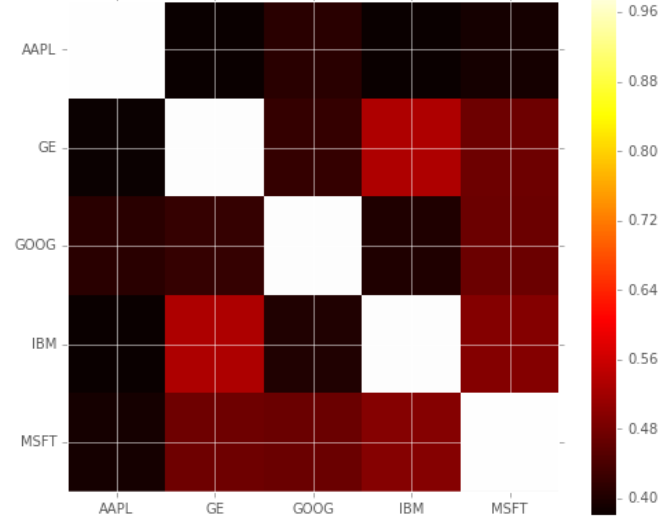### 股票回报率和风险

plt.scatter(retscomp.mean(), retscomp.std())
plt.xlabel('Expected returns')

plt.ylabel('Risk')

for label, x, y in zip(retscomp.columns, retscomp.mean(), retscomp.std()):

​    plt.annotate(label,xy = (x, y), xytext = (20, -20),
​       textcoords = 'offset points', ha = 'right', va = 'bottom',
​       bbox = dict(boxstyle = 'round,pad=0.5', fc = 'yellow', alpha = 0.5),
​       arrowprops = dict(arrowstyle = '->', connectionstyle = 'arc3,rad=0'))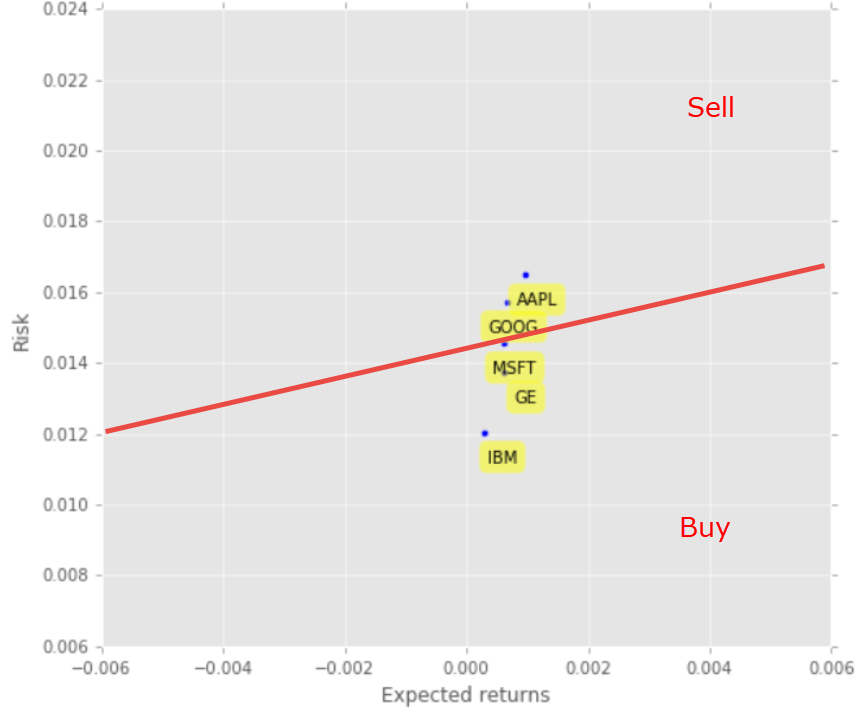## 预测股票价格

### 特征工程

dfreg = df.loc[:,['Adj Close','Volume']]

dfreg['HL_PCT'] = (df['High'] — df['Low']) / df['Close'] * 100.0

dfreg['PCT_change'] = (df['Close'] — df['Open']) / df['Open'] * 100.0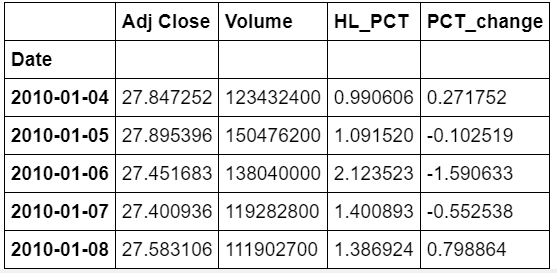### 预处理和交叉验证

1.删除缺失值

3.缩放 X ，使每个样本都可以具有相同的线性回归分布。

4.最后，我们要找到近期 X 和早期 X （用于训练）的数据序列，用于模型生成和评估。

5.分离标签并标识为 Y。

6.分别通过交叉验证训练模型和测试

# Drop missing value
dfreg.fillna(value=-99999, inplace=True)

# We want to separate 1 percent of the data to forecast
forecast_out = int(math.ceil(0.01 * len(dfreg)))

# Separating the label here, we want to predict the AdjClose

dfreg['label'] = dfreg[forecast_col].shift(-forecast_out)

X = np.array(dfreg.drop(['label'], 1))

# Scale the X so that everyone can have the same distribution for linear regression
X = preprocessing.scale(X)

# Finally We want to find Data Series of late X and early X (train) for model generation and evaluation
X_lately = X[-forecast_out:]
X = X[:-forecast_out]

# Separate label and identify it as y
y = np.array(dfreg['label'])
y = y[:-forecast_out]


### 模型生成-预测过程有意思的地方开始了

from sklearn.linear_model import LinearRegression
from sklearn.neighbors import KNeighborsRegressor
from sklearn.linear_model import Ridge
from sklearn.preprocessing import PolynomialFeatures
from sklearn.pipeline import make_pipeline


### 简单线性分析和二次判别分析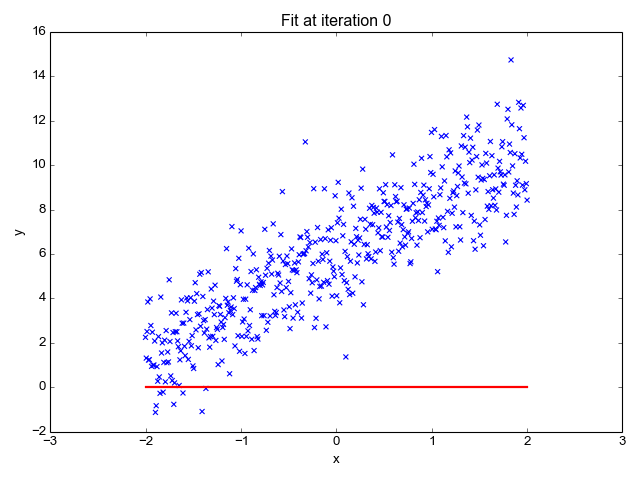A beginner’s guide to Linear Regression in Python with Scikit-Learn https://towardsdatascience.com/a-beginners-guide-to-linear-regression-in-python-with-scikit-learn-83a8f7ae2b4f

# Linear regression
clfreg = LinearRegression(n_jobs=-1)
clfreg.fit(X_train, y_train)

clfpoly2 = make_pipeline(PolynomialFeatures(2), Ridge())
clfpoly2.fit(X_train, y_train)

clfpoly3 = make_pipeline(PolynomialFeatures(3), Ridge())
clfpoly3.fit(X_train, y_train)


### K最近邻(KNN)

KNN利用特征相似性来预测数据点的值。这保证了分配的新点与数据集中的点相似。为了找出相似点，我们提取这些点间的最小距离(例如：欧氏距离)。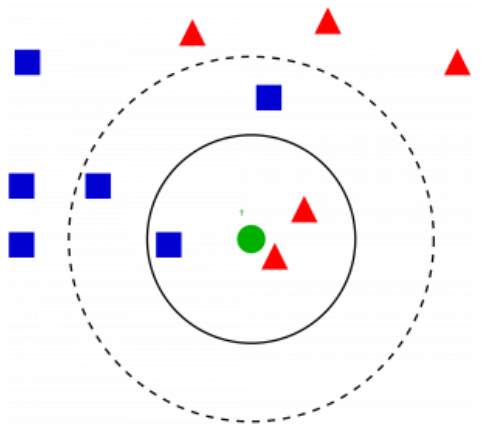Introduction to k-Nearest Neighbors: Simplified (with implementation in Python) https://www.analyticsvidhya.com/blog/2018/03/introduction-k-neighbours-algorithm-clustering/

# KNN Regression
clfknn = KNeighborsRegressor(n_neighbors=2)
clfknn.fit(X_train, y_train)


### 评估


confidencereg = clfreg.score(X_test, y_test)
confidencepoly2 = clfpoly2.score(X_test,y_test)
confidencepoly3 = clfpoly3.score(X_test,y_test)
confidenceknn = clfknn.score(X_test, y_test)

# results

('The linear regression confidence is ', 0.96399641826551985)

('The quadratic regression 2 confidence is ', 0.96492624557970319)

('The quadratic regression 3 confidence is ', 0.9652082834532858)

('The knn regression confidence is ', 0.92844658034790639)



forecast_set = clf.predict(X_lately)

dfreg['Forecast'] = np.nan

#result

(array([ 115.44941187,  115.20206522,  116.78688393,  116.70244946,

116.58503739,  115.98769407,  116.54315699,  117.40012338,

117.21473053,  116.57244657,  116.048717  ,  116.26444966,

115.78374093,  116.50647805,  117.92064806,  118.75581186,

​        118.82688731,  119.51873699]), 0.96234891774075604, 18)


## 绘制预测图

last_date = dfreg.iloc[-1].name

last_unix = last_date

next_unix = last_unix + datetime.timedelta(days=1)

for i in forecast_set:

next_date = next_unix
next_unix += datetime.timedelta(days=1)
dfreg.loc[next_date] = [np.nan for _ in range(len(dfreg.columns)-1)]+[i]

dfreg['Forecast'].tail(500).plot()
plt.legend(loc=4)
plt.xlabel('Date')
plt.ylabel('Price')
plt.show()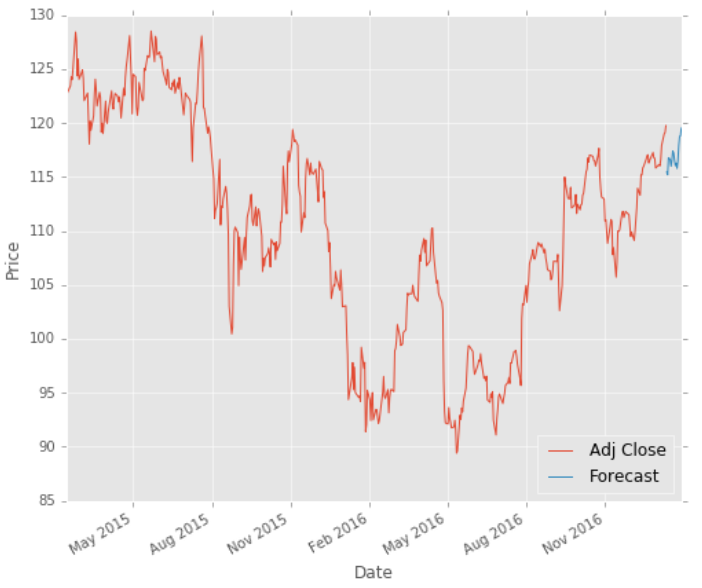## 未来的改进/挑战

• 分析经济定性因素，如新闻（新闻来源和情感分析）

• 分析经济定量因素，如某个国家的HPI、公司起源之间的经济不平等